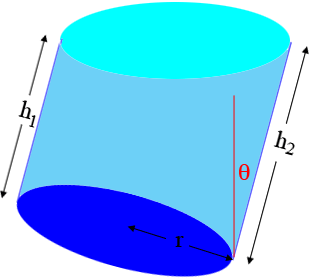SEARCH HOMEMath Central Quandaries & QueriesQuestion from Astri, a student: I have a problem determining the fluid level for tilted cylinder tank. Actually I've found some solution here for horizontal cylinder tank. What if the cylinder is tilted at a certain degree, for example 30 degree from the vertical axis. Thank you.Hi Astri,

I am not sure what you are looking for. The questions we have received for a horizontal tank ask for the volume of liquid in the tank when the height of the liquid is given. For example this is the question that Dave asked a while ago.

If you want the volume of liquid in a tilted cylindrical tank as indicated in the diagram below then the volume $V$ is given by

$V = \pi r^2 \times \frac{h_1 + h_2}{2} \mbox{ cubic units}$

where $h_1$ and $h_2$ are the shortest and longest measurements from the bottom to the top respectively.I assume that you know $h_2$ and the angle $\theta$ in degrees. If so then you can find $h_1$ by

$h_1 = h_2 - 2r \tan \theta .$

I hope this helps,
PennyMath Central is supported by the University of Regina and the Imperial Oil Foundation.